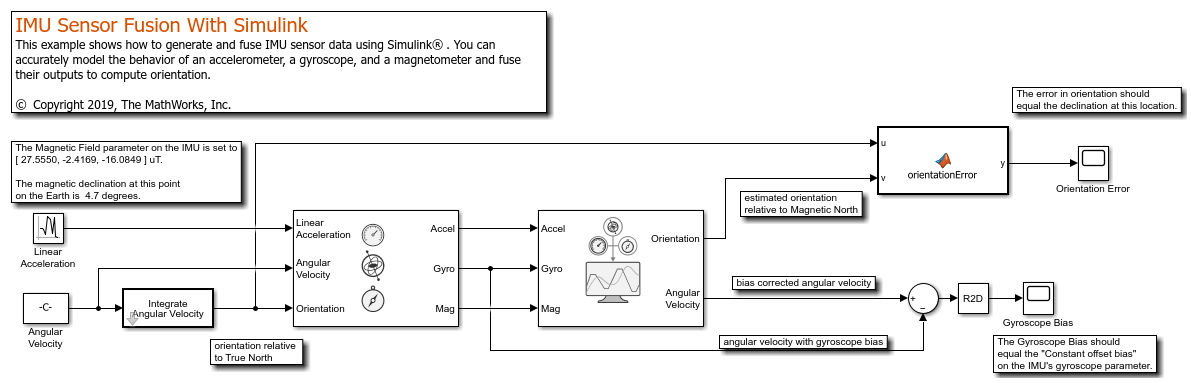# IMU Sensor Fusion with Simulink

This example shows how to generate and fuse IMU sensor data using Simulink®. You can accurately model the behavior of an accelerometer, a gyroscope, and a magnetometer and fuse their outputs to compute orientation.

### Inertial Measurement Unit

An inertial measurement unit (IMU) is a group of sensors consisting of an accelerometer measuring acceleration and a gyroscope measuring angular velocity. Frequently, a magnetometer is also included to measure the Earth's magnetic field. Each of these three sensors produces a 3-axis measurement, and these three measurements constitute a 9-axis measurement.

### Attitude Heading and Reference System

An Attitude Heading and Reference System (AHRS) takes the 9-axis sensor readings and computes the orientation of the device. This orientation is given relative to the NED frame, where N is the Magnetic North direction. The AHRS block in Simulink accomplishes this using an indirect Kalman filter structure.

Open the Simulink model that fuses IMU sensor data

```open_system('IMUFusionSimulinkModel'); ```### Inputs and Configuration

The inputs to the IMU block are the device's linear acceleration, angular velocity, and the orientation relative to the navigation frame. The orientation is of the form of a quaternion (a 4-by-1 vector in Simulink) or rotation matrix (a 3-by-3 matrix in Simulink) that rotates quantities in the navigation frame to the body frame. In this model, the angular velocity is simply integrated to create an orientation input. The angular velocity is in rad/s and the linear acceleration is in m/s^2. Because the AHRS has only one input related to translation (the accelerometer input), it cannot distinguish between gravity and linear acceleration. Therefore, the AHRS algorithm assumes that linear acceleration is a slowly varying white noise process. This is a common assumption for 9-axis fusion algorithms.

### True North vs Magnetic North

Magnetic field parameter on the IMU block dialog can be set to the local magnetic field value. Magnetic field values can be found on the NOAA website or using the `wrldmagm` function in the Aerospace Toolbox™. The magnetic field values on the IMU block dialog correspond the readings of a perfect magnetometer that is orientated to True North. Therefore, the orientation input to the IMU block is relative to the NED frame, where N is the True North direction. However, the AHRS filter navigates towards Magnetic North, which is typical for this type of filter. Therefore, the orientation input to the IMU and the estimated orientation at the output of the AHRS differ by the declination angle between True North and Magnetic North.

This simulation is setup forlatitude andlongitude. The magnetic field at this location is set as [27.5550, -2.4169, -16.0849] microtesla in the IMU block. The declination at this location is about### Simulation

Simulate the model. The IMU input orientation and the estimated output orientation of the AHRS are compared using quaternion distance. This is preferable compared to differencing the Euler angle equivalents, considering the Euler angle singularities.

```sim('IMUFusionSimulinkModel'); ```

### Estimated Orientation

The difference in estimated vs true orientation should be nearly, which is the declination at this latitude and longitude.

### Gyroscope Bias

The second output of the AHRS filter is the bias-corrected gyroscope reading. In the IMU block, the gyroscope was given a bias of 0.0545 rad/s or 3.125 deg/s, which should match the steady state value in the `Gyroscope Bias` scope block.

### Further Exercises

By varying the parameters on the IMU, you should see a corresponding change in orientation on the output of the AHRS. You can set the parameters on the IMU block to match a real IMU datasheet and tune the AHRS parameters to meet your requirements.